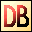DelphiBasicsRoundFunction Rounds a floating point number to an integer System unit
 function Round ( const Number : Extended ) : Int64;
Description
The Round function rounds a floating point Number to an Integer value.

The rounding uses Bankers rules, where an exact half value causes a rounding to an even number:

 12.4 rounds to 12 12.5 rounds to 12 // Round down to even 12.6 rounds to 13 13.4 rounds to 13 13.5 rounds to 14 // Round up to even 13.6 rounds to 14
Notes
The Trunc function does the same, but returns the integer in an Integer value.
Related commands
 Frac The fractional part of a floating point number Int The integer part of a floating point number as a float Trunc The integer part of a floating point numberDownload this web site as a Windows program.